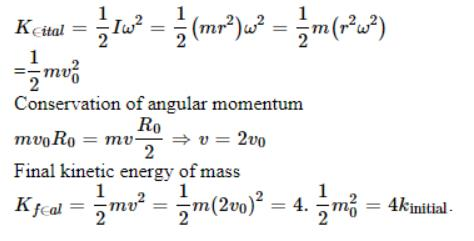Courses

# Test: System Of Particles And Rotational Motion 2 - From Past 28 Years Questions

## 30 Questions MCQ Test Physics 28 Years Past year papers for NEET/AIPMT Class 11 | Test: System Of Particles And Rotational Motion 2 - From Past 28 Years Questions

Description
This mock test of Test: System Of Particles And Rotational Motion 2 - From Past 28 Years Questions for NEET helps you for every NEET entrance exam. This contains 30 Multiple Choice Questions for NEET Test: System Of Particles And Rotational Motion 2 - From Past 28 Years Questions (mcq) to study with solutions a complete question bank. The solved questions answers in this Test: System Of Particles And Rotational Motion 2 - From Past 28 Years Questions quiz give you a good mix of easy questions and tough questions. NEET students definitely take this Test: System Of Particles And Rotational Motion 2 - From Past 28 Years Questions exercise for a better result in the exam. You can find other Test: System Of Particles And Rotational Motion 2 - From Past 28 Years Questions extra questions, long questions & short questions for NEET on EduRev as well by searching above.
QUESTION: 1

### A solid sphere of radius R is placed on a smooth horizontal surface. A horizontal force F is applied at height h from the lowest point. For the maximum acceleration of the centre of mass, 

Solution:

As friction is absent at the point of contact,

Accelerati on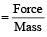It is independent of h

QUESTION: 2

### A boy suddenly comes and sits on a circular rotating table. What will remain conserved? 

Solution:

As net torque applied is zero. Hence,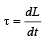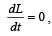, L = constant.

L (angular momentum) remains conserved.

QUESTION: 3

### A ball rolls without slipping. The radius of gyration of the ball about an axis passing through its centre of mass is K. If radius of the ball be R, then the fraction of total energy associated with its rotational energy will be [2003

Solution:

Rotational energy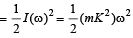Linear kinetic energy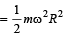∴ Required fraction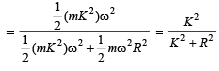QUESTION: 4

A solid cylinder of mass M and radius R rolls without slipping down an inclined plane of length L and height h. What is the speed of its centre of mass when the cylinder reaches its bottom ? 

Solution:

Applying principle of conservation of total mechanical energy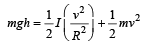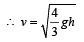QUESTION: 5

A thin circular ring of mass M and radius r is rotating about its axis with a constant angular velocity ω. Four objects each of mass m, are kept gently to the opposite ends of two perpendicular diameters of the ring. The angular velocity of the ring will be 

Solution:

Applying conservation law of angular momentum, I1ω1 = I2ω2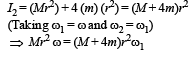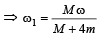QUESTION: 6

A wheel having moment of inertia 2 kg-m2 about its vertical axis, rotates at the rate of 60 rpm about this axis. The torque which can stop the wheel’s rotation in one minute would be 

Solution: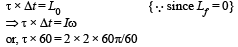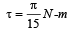QUESTION: 7

Consider a system of two particles having masses m1 and m2 . If the particle of mass m1 is pushed towards the centre of mass of particles through a distance d, by what distance would the particle of mass m2 move so as to keep the centre of mass of particles at the original position? 

Solution: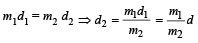QUESTION: 8

The ratio of the radii of gyration of a circular disc about a tangential axis in the plane of the disc and of a circular ring of the same radius about a tangential axis in the plane of the ring is 

Solution: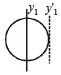Circular disc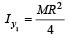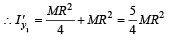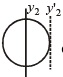Circular ring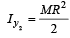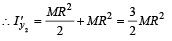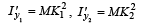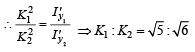QUESTION: 9

A r ound disc of moment of in ertia I2 about its axis perpendicular to its plane and passing through its centre is placed over another   disc of moment of inertia I1 rotating with an angular velocity ω about the same axis. The final angular velocity of the combin ation of discs is 

Solution:

Angular momentum will be conserved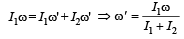QUESTION: 10

Three particles, each of mass m gram, are situated at the vertices of an equilateral triangle ABC of side l cm (as shown in the figure). The moment of inertia of the system about a line AX perpendicular to AB and in the plane of ABC, in gram-cm2 units will be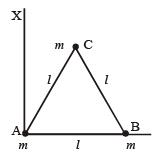Solution: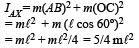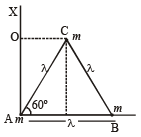QUESTION: 11

The moment of inertia of a uniform circular disc of radius R and mass M about an axis passing from the edge of the disc and normal to the disc is : [2 00 5]

Solution:

M.I. of a uniform circular disc of radius ‘R’ and mass ‘M’ about an axis passing though C.M. and normal to the disc  is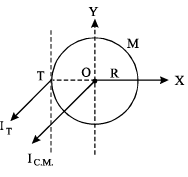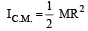From parallel axis theorem,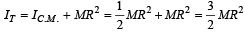QUESTION: 12

​Two bodies have th eir momen ts of in er tia I and 2I respectively about their axis of rotation. If their kinetic energies of rotation are equal, their angular momenta will be in the ratio

Solution: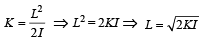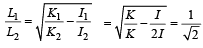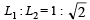QUESTION: 13

A drum of radius R and mass M, rolls down without slipping along an inclined plane of angle θ. The frictional force 

Solution:

Net work done by frictional force when drum rolls down without slipping is zero.
Wnet = 0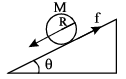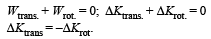i.e., converts translation energy to rotational energy.

QUESTION: 14

The moment of inertia of a uniform circular disc of radius R and mass M about an axis touching the disc at its diameter and normal to the disc is

Solution:

Moment of Inertia of a disc about an axis perpendicular to its face & passing through its centre is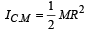Now, given axis is parallel to the axis given above,  so, applying theorem of parallel axis.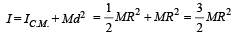QUESTION: 15

A tube of length L is filled completely with an incompressible liquid of mass M and closed at both the ends. The tube is then rotated in a horizontal plane about one of its ends with a uniform angular velocity ω. The force exerted by the liquid at the other end is 

Solution: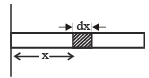Rotation will create centrifugal force due to which pressure will be created at the other end. Now, this centrifugal force will depend on distance of the liquid from the axis as centrifugal force = mω2r.
Let us consider a small part dx of the liquid column which is at a distance of x. Let linear density of liquid be ρ.
Mass of the liquid in dx length = dx.A.ρ (A is cross sectional area of tube) Centrifugal force = dxAρ × ω2x Centrifugal force due to whole of liquid length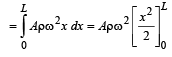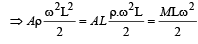QUESTION: 16

A wheel has angular acceleration of 3.0 rad/sec2 and an initial angular speed of 2.00 rad/sec. In a time of 2 sec it has rotated through an angle (in radian) of 

Solution:

Since,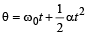Where α is angular aceleration, ωo is the initial angular speed. t = 2s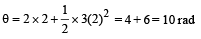QUESTION: 17

A uniform rod AB of length ℓ, and mass m is free to rotate about point A. The rod is released from rest in the horizontal position. Given that the moment of inertia of the rod about A is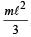, the initial angular acceleration of the rod will be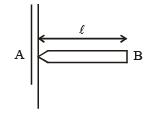Solution: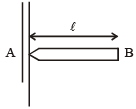Weight of the rod will produce torque,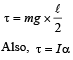where, I is the moment of inertia =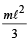and α is the angular acceleration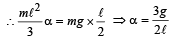QUESTION: 18

A particle of mass m moves in the XY plane with a velocity v along the straight line AB. If the angular momentum of the particle with respect to origin O is LA when it is at A and LB when it is at B, then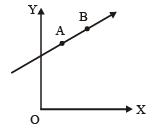Solution:

Angular momentum = Linear momentum × distance of line of action of linear momentum about the origin.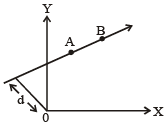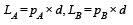As linear momenta are pA and pB  equal, therefore, LA = LB.

QUESTION: 19

The ratio of the radii or gyration of a circular disc to that of a circular ring, each of same mass and radius, around their respective axes is:

Solution:

The radius of gyration of a circular disc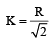The radius of gyration of a circular ring K' = R (Since both have the same mass and radius)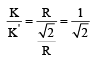QUESTION: 20

A thin rod of length L and mass M is bent at its midpoint into two halves so that the angle between them is 90°. The moment of inertia of the bent rod about an axis passing through the bending point and perpendicular to the plane defined by the two halves of the rod is:   

Solution:

Mass of each part = M/2 Length of each part = L/2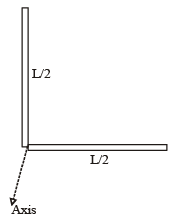Total M.I. = Sum of M.I.s of both parts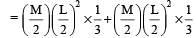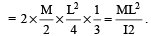QUESTION: 21

A t hin circular ring of mass M an d radius R is rotating in a horizontal plane about an axis vertical to its plane with a constant angular velocity ω. If two objects each of mass m be attached gently to the opposite ends of a diameter of the ring, the ring will then rotate with an angular velocity: 

Solution:

In absence of exter nal torque, L = Iω  = constant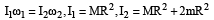(Moment of inertia of a thin circular ring about an axis vertical to its plane = MR2)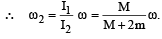QUESTION: 22

If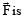the force acting on a particle having position vector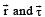be the tor que of this force about the origin, then: 

Solution: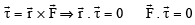Since,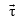is perpendicular to the plane of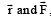, h ence the dot product ofwithis zero.

QUESTION: 23

Four identical th in rods each of mass M and length l, form a square frame. Moment of inertia of this frame about an axis through the centre of the square and perpendicular to its plane is :   

Solution:

Moment of in ertia of a thin rod of length l about an axis passing through centre and perpendicular to the rod =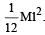Thus moment of inertia of the frame.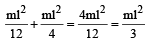Total  M.I.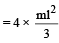QUESTION: 24

Two bodies of mass 1 kg and 3 kg have position vectors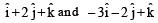ˆ respectively. The centre of mass of this system has a position vector: 

Solution:

The position vector of the centre of mass of two particle system is given by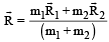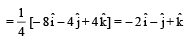QUESTION: 25

A circular disk of moment of inertia It is rotating in a horizontal plane, its symmetry axis, with a constant angular speed ωi . Another disk of moment of inertia Ib is dropped coaxially onto the rotating disk. Initially the second disk has zero angular speed. Eventually both the disks rotate with a constant angular speed ωf . The energy lost by the initially rotating disk to friction is:


Solution:

By conservation of angular momentum,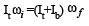where ωf is the final angular velocity ofdisks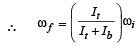Loss in K.E., ΔK = Initial K.E. – Final K.E.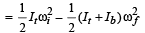⇒ ΔK =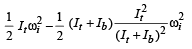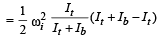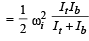QUESTION: 26

Two particles which are initially at rest, move towards each other under the action of their internal attraction. If their speeds are v and 2v at any instant, then the speed of centre of mass of the system will be: 

Solution:

If n o extern al for ce actson a system of particles, the centre of mass remains at rest.
So, speed of centre of mass is zero.

QUESTION: 27

A gramophone recor d is revolving with an angular velocity ω. A coin is placed at a distance r from the centre of the record. The static coefficient of friction is ω. The coin will revolve with the record if: 

Solution:

For the coin to revolve with the record, centrifugal force ≤ frictional force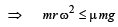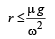QUESTION: 28

The instantaneous angular position of a point on a rotating wheel is given by the equation θ(t) = 2t3 – 6t2. The torque on the wheel becomes zero at

Solution:

When angular acceleration (α) is zero then torque on the wheel becomes zero. θ(t) = 2t3 – 6t2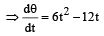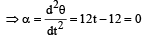∴  t = 1 sec.

QUESTION: 29

The moment of inertia of a thin uniform rod of mass M and length L about an axis passing through its midpoint and perpendicular to its length is I0. Its moment of inertia about an axis passing through one of its ends and perpendicular to its length is 

Solution:

By theorem of parallel axes,QUESTION: 30

A small mass attached to a string rotates on frictionless table top as shown. If the tension in the string is increased by pulling the string causing the radius of the circular motion to decrease by a factor of 2, the kinetic energy of the mass will [2011M]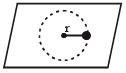Solution: